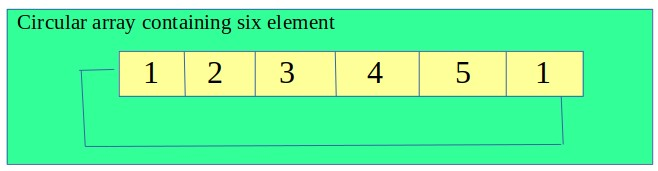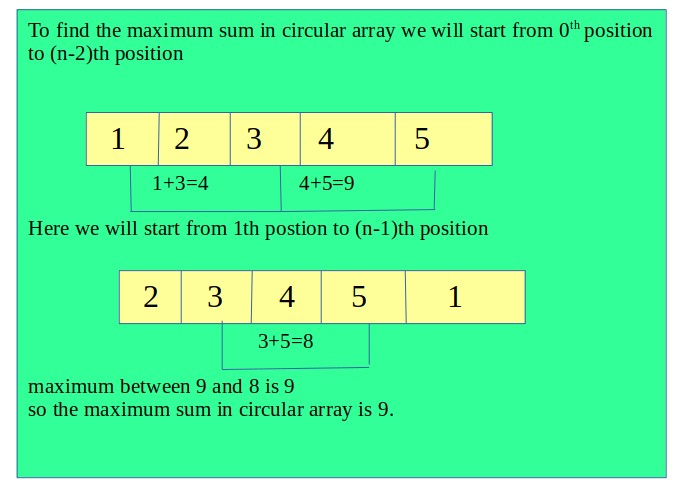# Maximum sum in circular array such that no two elements are adjacent

Given a circular array containing of positive integers value. The task is to find the maximum sum of a subsequence with the constraint that no 2 numbers in the sequence should be adjacent in the array.

Examples:

```Input: circular arr = {1, 2, 3, 1}
Output : 4
subsequence will be(1, 3), hence 1 + 3 = 4

Input: circular arr = {1, 2, 3, 4, 5, 1}
Output: 9
subsequence will be(1, 3, 5), hence 1 + 3 + 5 = 9
```

## Recommended: Please try your approach on {IDE} first, before moving on to the solution.

Approach The problem can be solved using DP. An approach has already been discussed in this post, but it for an array. We can treat the circular subarray a two arrays one from (0th to n-2-th) and (1st to n-1-th) index, and use the approach used in the previous post. The maximum sum returned by both will be the answer.Below is the implementation of the above approach.

## C++

 `// CPP program to find maximum sum in a circular array  ` `// such that no elements are adjacent in the sum. ` `#include ` `using` `namespace` `std; ` ` `  `// Function to calculate the sum ` `// from 0th position to(n-2)th position ` `int` `maxSum1(``int` `arr[], ``int` `n) ` `{ ` `    ``int` `dp[n]; ` `    ``int` `maxi = 0; ` ` `  `    ``for` `(``int` `i = 0; i < n - 1; i++) { ` ` `  `        ``// copy the element of original array to dp[] ` `        ``dp[i] = arr[i]; ` ` `  `        ``// find the maximum element in the array ` `        ``if` `(maxi < arr[i]) ` `            ``maxi = arr[i]; ` `    ``} ` ` `  `    ``// start from 2nd to n-1th pos ` `    ``for` `(``int` `i = 2; i < n - 1; i++) { ` ` `  `        ``// traverse for all pairs ` `        ``// bottom-up approach ` `        ``for` `(``int` `j = 0; j < i - 1; j++) { ` ` `  `            ``// dp-condition ` `            ``if` `(dp[i] < dp[j] + arr[i]) { ` `                ``dp[i] = dp[j] + arr[i]; ` ` `  `                ``// find maximum sum ` `                ``if` `(maxi < dp[i]) ` `                    ``maxi = dp[i]; ` `            ``} ` `        ``} ` `    ``} ` ` `  `    ``// return the maximum ` `    ``return` `maxi; ` `} ` ` `  `// Function to find the maximum sum ` `// from 1st position to n-1-th position ` `int` `maxSum2(``int` `arr[], ``int` `n) ` `{ ` `    ``int` `dp[n]; ` `    ``int` `maxi = 0; ` ` `  `    ``for` `(``int` `i = 1; i < n; i++) { ` `        ``dp[i] = arr[i]; ` ` `  `        ``if` `(maxi < arr[i]) ` `            ``maxi = arr[i]; ` `    ``} ` ` `  `    ``// Traverse from third to n-th pos ` `    ``for` `(``int` `i = 3; i < n; i++) { ` ` `  `        ``// bootom-up approach ` `        ``for` `(``int` `j = 1; j < i - 1; j++) { ` ` `  `            ``// dp condition ` `            ``if` `(dp[i] < arr[i] + dp[j]) { ` `                ``dp[i] = arr[i] + dp[j]; ` ` `  `                ``// find max sum ` `                ``if` `(maxi < dp[i]) ` `                    ``maxi = dp[i]; ` `            ``} ` `        ``} ` `    ``} ` ` `  `    ``// return max ` `    ``return` `maxi; ` `} ` ` `  `int` `findMaxSum(``int` `arr[], ``int` `n) ` `{ ` `   ``return` `max(maxSum1(arr, n), maxSum2(arr, n)); ` `} ` ` `  `// Driver Code ` `int` `main() ` `{ ` `    ``int` `arr[] = { 1, 2, 3, 1 }; ` `    ``int` `n = ``sizeof``(arr)/``sizeof``(arr); ` `    ``cout << findMaxSum(arr, n); ` `    ``return` `0; ` `} `

## Java

 `// Java  program to find maximum sum in a circular array  ` `// such that no elements are adjacent in the sum.  ` ` `  `import` `java.io.*; ` ` `  `class` `GFG { ` `     `  `     `  `// Function to calculate the sum  ` `// from 0th position to(n-2)th position  ` `static` `int` `maxSum1(``int` `arr[], ``int` `n)  ` `{  ` `    ``int` `dp[]=``new` `int``[n]; ` `    ``int` `maxi = ``0``;  ` ` `  `    ``for` `(``int` `i = ``0``; i < n - ``1``; i++) {  ` ` `  `        ``// copy the element of original array to dp[]  ` `        ``dp[i] = arr[i];  ` ` `  `        ``// find the maximum element in the array  ` `        ``if` `(maxi < arr[i])  ` `            ``maxi = arr[i];  ` `    ``}  ` ` `  `    ``// start from 2nd to n-1th pos  ` `    ``for` `(``int` `i = ``2``; i < n - ``1``; i++) {  ` ` `  `        ``// traverse for all pairs  ` `        ``// bottom-up approach  ` `        ``for` `(``int` `j = ``0``; j < i - ``1``; j++) {  ` ` `  `            ``// dp-condition  ` `            ``if` `(dp[i] < dp[j] + arr[i]) {  ` `                ``dp[i] = dp[j] + arr[i];  ` ` `  `                ``// find maximum sum  ` `                ``if` `(maxi < dp[i])  ` `                    ``maxi = dp[i];  ` `            ``}  ` `        ``}  ` `    ``}  ` ` `  `    ``// return the maximum  ` `    ``return` `maxi;  ` `}  ` ` `  `// Function to find the maximum sum  ` `// from 1st position to n-1-th position  ` `static` `int` `maxSum2(``int` `arr[], ``int` `n)  ` `{  ` `    ``int` `dp[]=``new` `int``[n]; ` `    ``int` `maxi = ``0``;  ` ` `  `    ``for` `(``int` `i = ``1``; i < n; i++) {  ` `        ``dp[i] = arr[i];  ` ` `  `        ``if` `(maxi < arr[i])  ` `            ``maxi = arr[i];  ` `    ``}  ` ` `  `    ``// Traverse from third to n-th pos  ` `    ``for` `(``int` `i = ``3``; i < n; i++) {  ` ` `  `        ``// bootom-up approach  ` `        ``for` `(``int` `j = ``1``; j < i - ``1``; j++) {  ` ` `  `            ``// dp condition  ` `            ``if` `(dp[i] < arr[i] + dp[j]) {  ` `                ``dp[i] = arr[i] + dp[j];  ` ` `  `                ``// find max sum  ` `                ``if` `(maxi < dp[i])  ` `                    ``maxi = dp[i];  ` `            ``}  ` `        ``}  ` `    ``}  ` ` `  `    ``// return max  ` `    ``return` `maxi;  ` `}  ` ` `  `static` `int` `findMaxSum(``int` `arr[], ``int` `n)  ` `{  ` `    ``int` `t=Math.max(maxSum1(arr, n), maxSum2(arr, n)); ` `    ``return`  `t; ` `}  ` ` `  `// Driver Code  ` `    ``public` `static` `void` `main (String[] args) { ` `         `  `        ``int` `arr[] = { ``1``, ``2``, ``3``, ``1` `};  ` `        ``int` `n = arr.length;  ` `        ``System.out.println(findMaxSum(arr, n));  ` `         `  `    ``} ` `} `

## Python 3

 `# Python 3 program to find maximum sum  ` `# in a circular array such that no  ` `# elements are adjacent in the sum. ` ` `  `# Function to calculate the sum from ` `# 0th position to(n-2)th position ` `def` `maxSum1(arr, n): ` ` `  `    ``dp ``=` `[``0``] ``*` `n ` `    ``maxi ``=` `0` ` `  `    ``for` `i ``in` `range``(n ``-` `1``): ` ` `  `        ``# copy the element of original  ` `        ``# array to dp[] ` `        ``dp[i] ``=` `arr[i] ` ` `  `        ``# find the maximum element in the array ` `        ``if` `(maxi < arr[i]): ` `            ``maxi ``=` `arr[i] ` ` `  `    ``# start from 2nd to n-1th pos ` `    ``for` `i ``in` `range``(``2``, n ``-` `1``): ` ` `  `        ``# traverse for all pairs bottom-up ` `        ``# approach ` `        ``for` `j ``in` `range``(i ``-` `1``) : ` ` `  `            ``# dp-condition ` `            ``if` `(dp[i] < dp[j] ``+` `arr[i]): ` `                ``dp[i] ``=` `dp[j] ``+` `arr[i] ` ` `  `                ``# find maximum sum ` `                ``if` `(maxi < dp[i]): ` `                    ``maxi ``=` `dp[i] ` ` `  `    ``# return the maximum ` `    ``return` `maxi ` ` `  `# Function to find the maximum sum ` `# from 1st position to n-1-th position ` `def` `maxSum2(arr, n): ` ` `  `    ``dp ``=` `[``0``] ``*` `n ` `    ``maxi ``=` `0` ` `  `    ``for` `i ``in` `range``(``1``, n): ` `        ``dp[i] ``=` `arr[i] ` ` `  `        ``if` `(maxi < arr[i]): ` `            ``maxi ``=` `arr[i] ` ` `  `    ``# Traverse from third to n-th pos ` `    ``for` `i ``in` `range``(``3``, n): ` ` `  `        ``# bootom-up approach ` `        ``for` `j ``in` `range``(``1``, i ``-` `1``) : ` ` `  `            ``# dp condition ` `            ``if` `(dp[i] < arr[i] ``+` `dp[j]): ` `                ``dp[i] ``=` `arr[i] ``+` `dp[j] ` ` `  `                ``# find max sum ` `                ``if` `(maxi < dp[i]): ` `                    ``maxi ``=` `dp[i] ` ` `  `    ``# return max ` `    ``return` `maxi ` ` `  `def` `findMaxSum(arr, n): ` `    ``return` `max``(maxSum1(arr, n), maxSum2(arr, n)) ` ` `  `# Driver Code ` `if` `__name__ ``=``=` `"__main__"``: ` `     `  `    ``arr ``=` `[ ``1``, ``2``, ``3``, ``1` `] ` `    ``n ``=` `len``(arr) ` `    ``print``(findMaxSum(arr, n)) ` ` `  `# This code is contributed by ita_c `

## C#

 `// C# program to find maximum sum ` `// in a circular array such that  ` `// no elements are adjacent in the sum.  ` `using` `System; ` ` `  `class` `GFG ` `{ ` `// Function to calculate the sum  ` `// from 0th position to(n-2)th position  ` `static` `int` `maxSum1(``int` `[]arr, ``int` `n)  ` `{  ` `    ``int` `[]dp = ``new` `int``[n];  ` `    ``int` `maxi = 0;  ` ` `  `    ``for` `(``int` `i = 0; i < n - 1; i++) ` `    ``{  ` ` `  `        ``// copy the element of original ` `        ``// array to dp[]  ` `        ``dp[i] = arr[i];  ` ` `  `        ``// find the maximum element  ` `        ``// in the array  ` `        ``if` `(maxi < arr[i])  ` `            ``maxi = arr[i];  ` `    ``}  ` ` `  `    ``// start from 2nd to n-1th pos  ` `    ``for` `(``int` `i = 2; i < n - 1; i++)  ` `    ``{  ` ` `  `        ``// traverse for all pairs  ` `        ``// bottom-up approach  ` `        ``for` `(``int` `j = 0; j < i - 1; j++)  ` `        ``{  ` ` `  `            ``// dp-condition  ` `            ``if` `(dp[i] < dp[j] + arr[i]) ` `            ``{  ` `                ``dp[i] = dp[j] + arr[i];  ` ` `  `                ``// find maximum sum  ` `                ``if` `(maxi < dp[i])  ` `                    ``maxi = dp[i];  ` `            ``}  ` `        ``}  ` `    ``}  ` ` `  `    ``// return the maximum  ` `    ``return` `maxi;  ` `}  ` ` `  `// Function to find the maximum sum  ` `// from 1st position to n-1-th position  ` `static` `int` `maxSum2(``int` `[]arr, ``int` `n)  ` `{  ` `    ``int` `[]dp = ``new` `int``[n];  ` `    ``int` `maxi = 0;  ` ` `  `    ``for` `(``int` `i = 1; i < n; i++)  ` `    ``{  ` `        ``dp[i] = arr[i];  ` ` `  `        ``if` `(maxi < arr[i])  ` `            ``maxi = arr[i];  ` `    ``}  ` ` `  `    ``// Traverse from third to n-th pos  ` `    ``for` `(``int` `i = 3; i < n; i++) ` `    ``{  ` ` `  `        ``// bootom-up approach  ` `        ``for` `(``int` `j = 1; j < i - 1; j++) ` `        ``{  ` ` `  `            ``// dp condition  ` `            ``if` `(dp[i] < arr[i] + dp[j]) ` `            ``{  ` `                ``dp[i] = arr[i] + dp[j];  ` ` `  `                ``// find max sum  ` `                ``if` `(maxi < dp[i])  ` `                    ``maxi = dp[i];  ` `            ``}  ` `        ``}  ` `    ``}  ` ` `  `    ``// return max  ` `    ``return` `maxi;  ` `}  ` ` `  `static` `int` `findMaxSum(``int` `[]arr, ``int` `n)  ` `{  ` `    ``int` `t = Math.Max(maxSum1(arr, n),  ` `                     ``maxSum2(arr, n));  ` `    ``return` `t;  ` `}  ` ` `  `// Driver Code  ` `static` `public` `void` `Main () ` `{ ` `    ``int` `[]arr = { 1, 2, 3, 1 };  ` `    ``int` `n = arr.Length;  ` `    ``Console.WriteLine(findMaxSum(arr, n));  ` `} ` `} ` ` `  `// This code is contributed ` `// by Sach_Code `

## PHP

 ` `

Output:

```4
```

Time Complexity: O(N^2)

GeeksforGeeks has prepared a complete interview preparation course with premium videos, theory, practice problems, TA support and many more features. Please refer Placement 100 for details

My Personal Notes arrow_drop_upCheck out this Author's contributed articles.

If you like GeeksforGeeks and would like to contribute, you can also write an article using contribute.geeksforgeeks.org or mail your article to contribute@geeksforgeeks.org. See your article appearing on the GeeksforGeeks main page and help other Geeks.

Please Improve this article if you find anything incorrect by clicking on the "Improve Article" button below.